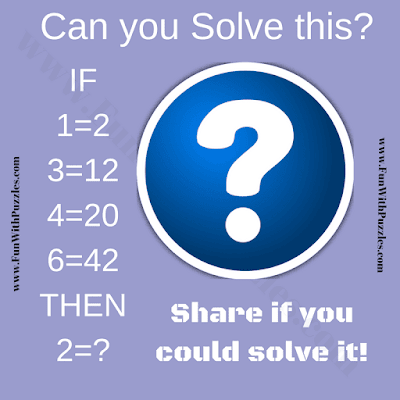Here is a very interesting logical question. In this Puzzle Image, there are some Mathematical equations which follow some logical pattern. Can you decode the logical reasoning for these equations and solve this Logic Question quickly?Can you solve this Logic Question?

The answer to this "Logic Question Image Puzzle", can be viewed by clicking on the button. Please do give your best try before looking at the answer.